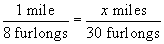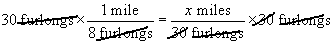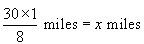Question from: Jennifer If there are 8 furlongs in a mile, how many miles are there in 30 furlongs? Hi Jennifer. This is a ratio (also known as a fraction). To solve this problem, we can rewrite the two parts of the question (separated by the comma) with furlongs and miles in the same order: If one MILE is 8 FURLONGS, how many MILES are in 30 FURLONGS? Now we can write this in algebraic symbols, we'll call the number of miles we are trying to find x:In algebraic equations like this, whatever we do to one side we must do to the other side. We want to get x miles alone on one side of the equation or the other. If we multiply both sides by 30 furlongs, then we can cancel the 30 and the furlongs from the right side and simplify the left side as well:This becomes:Can you solve it from here? Hope this helps, Stephen La Rocque. >